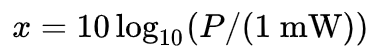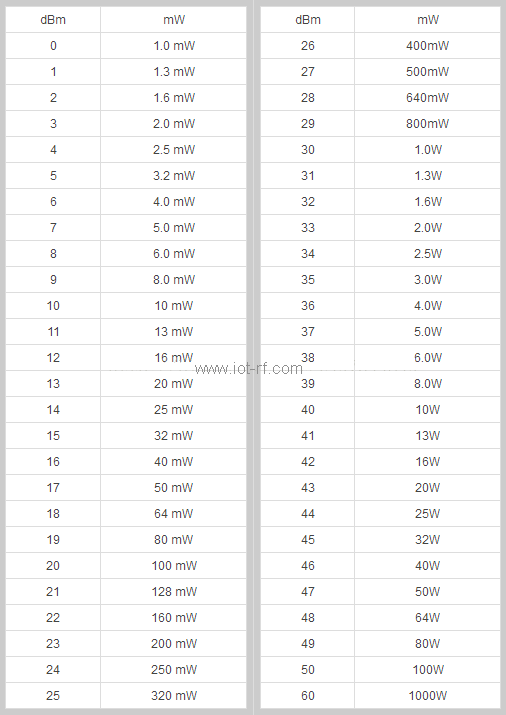You are here: / / / What is dBm? What does it have to do with the power unit mW?

# What is dBm? What does it have to do with the power unit mW?

Views:11     Author:James Wu     Publish Time: 2020-09-27      Origin:Site

What is dBm?

dBm means decibel milliwatt, full name in English is "decibel relative to one milliwatt", which refers to the absolute value of power.

As we may know, the output capability of the transmitter/amplifier is generally measured by power/level (dBm), and the unit is normally W, mW, dBm. While dBm is the absolute "power/level" expressed in decibels with 1mw as the reference value. The reason why the receiving sensitivity of a wireless module does not use the concept of "Watt" is because it is too small. For example, an RF module's receiving sensitivity is -90dBm, which is about 0.000000001mW;

The formula for converting arbitrary power P (mW) to X (dBm) is as follows:For example:

If the transmit power is 1mW, the converted value in dBm unit should be:

10 lg (1mW/1mW) = 0dBm;

If the transmit power is 40W, the converted value in dBm unit should be:

10log(40000mw/1mw) = 46dBm (Note: 1W = 1000mw)

What is db? What does it have to do with dBm?

db is a pure counting unit, representing a relative value, which can be understood as having no unit dimension, it must be the result of comparison; and dbm is the result of comparing the measured value with 1mw, which is an absolute value.

Examples are as follows:

If the absolute power measured by the power meter is X (mw), then the unit of dbm should be Y=10 * log (X/1mw). If this time we connect another transmission line where the power is measured, and test again from the end to get a power Q (mw), which can be calculated as Z (dbm), and the additional loss caused by this section of transmission line and joints can be easily calculated as Y-Z (db)

When calculating how many dB the power of A is greater or less than B, it can be calculated according to the formula 10lg A/B

Examples are as follows:

The power of A is twice the power of B, then 10lg (A/B) = 10 lg 2 = 3dB, that is, the power of A is 3dB greater than the power of B;

If the power of A is 46dBm and the power of B is 40dBm, it can be said that A is 6dB greater than B;

How to convert mw and dBm?

In the wireless modules, the conversion reference of commonly used dBm and mW is as follows:

10dBm=10mW，13dBm=20mW，16dBm=40mW，  19dBm=80mW，27dBm=500mW=0.5W，30dBm=1W，33dBm=2W，

It can be seen from the above that when the power is expressed in dBm, adding/subtracting 3dBm is equivalent to multiplying/dividing the linear value by 2, that is, the relationship of 2 times. Because when 3dBm is converted into a linear value: linear Value = 10^(3 / 10) = 2mw.DreamLnk offers various Wireless modules, include Wireless Receiving Modules, RF Transmitter Modules, 2.4GHz RF front-end modules, RF Transceiver Modules, RF Modules with Enhanced Power (PA), welcome to inquire!

Get Free Samples

One-stop IOT Solution Provider